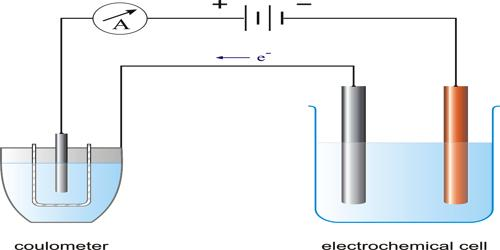ChemistryMicheal Faraday (1804) carried out a large number of experiments on electrolysis, and derived quantitative relationships between the quantity of electricity passed through the electrolyte and the amount of material liberated on the electrodes. The results of his experiments were expressed in the form of two laws. These are known as Faraday’s Law of electrolysis.

First Law of Electrolysis: The amount of substance dissolved from or liberated at an electrode is proportional to the quantity of electricity passed through the electrolyte solution.

The unit of the quantity of electricity is Coulomb. One Coulomb is the quantity of electricity when one ampere current flows through a conductor for one second. One coulomb is given the symbol C.If I ampere current flows for t seconds then the quantity of electricity passed is:

Q = (I x t) coulombs

Now if m is the mass in gram of substance liberated at an electrode by passing Q coulomb of electricity, according to Faraday’ first law:

m ∞ (I x t)

Where I is the current in Ampere (A) and ‘t’ is the time (in second) during which current was passed.

or, m = z x I x t

where z is a constant of proportionality and is known as the electrochemical equivalent of the substance. 1f I = 1 A and t = 1 second then, m = z. Thus,

The electrochemical equivalent is the amount of substance liberated when 1 A current is passed through the electrolyte for 1 second or wham one Coulomb of electricity is passed.

Second Law of Electrolysis: When the same quantity of electricity is passed through different electrolyte solutions of different substances, ‘equivalent masses’ of the elements are liberated at the electrodes.

These laws have been found to hold under all conditions, provided the current is carried entirely by the ions.